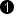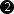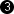## Operator Histogram

This operator calculates an intensity histogram from the input image at I and will output the results at output O. Depending on the option of the AutoSync parameter, the histogram is computed for each line or for each frame. The output is not a visualization of the results, but a list of the histogram values.

• AutoSync = EoF produces a histogram image of height 1 for the entire frame.
• AutoSync = EoL produces a histogram image of the input image height, i.e. a histogram is computed for each input image line separately.

The histogram itself consists of 2^(InputBitWidth) elements. Each element is the number of pixels for each tonal value. The first pixel at the histogram output is the number of pixels with tonal value zero.

Note that the input is blocked while the operator outputs its results. Therefore, small input images and or output for each image line reduce the bandwidth significantly.

Image Restrictions

• Empty Images

Empty images, i.e., images with no pixels, are not allowed.

### I/O Properties

Property Value
Operator Type M

Bit Width [1, 16] autoArithmetic unsigned as I
Parallelism any as I
Kernel Columns 1 as I
Kernel Rows 1 as I
Img Protocol VALT_IMAGE2D as I
Color Format VAF_GRAY as I
Color Flavor FL_NONE as I
Max. Img Width any autoMax. Img Height any autoThe output bit width depends on the max image dimension link properties and the setting of parameter AutoSync. For AutoSync = EoF the output bit width is For AutoSync = EoL the output bit width is The output bit width must not exceed 64 Bit. The output image width is determined by the input bit width. Each possible pixel value of the input image is represented by the pixels of the output image. For parameter AutoSync = EoF, the output image height is one. For parameter AutoSync = EoL, the output image height is equal to the input image height.

### Parameters

AutoSync
Type static parameter
Default EoL
Range {EoL, EoF}

This parameter selects whether to output a histogram every line (setting EoL) or every frame (setting EoF). Note the parameter's influence on the output bit width. The output bit width must not exceed 64 Bit.

### Examples of Use

The use of operator Histogram is shown in the following examples: